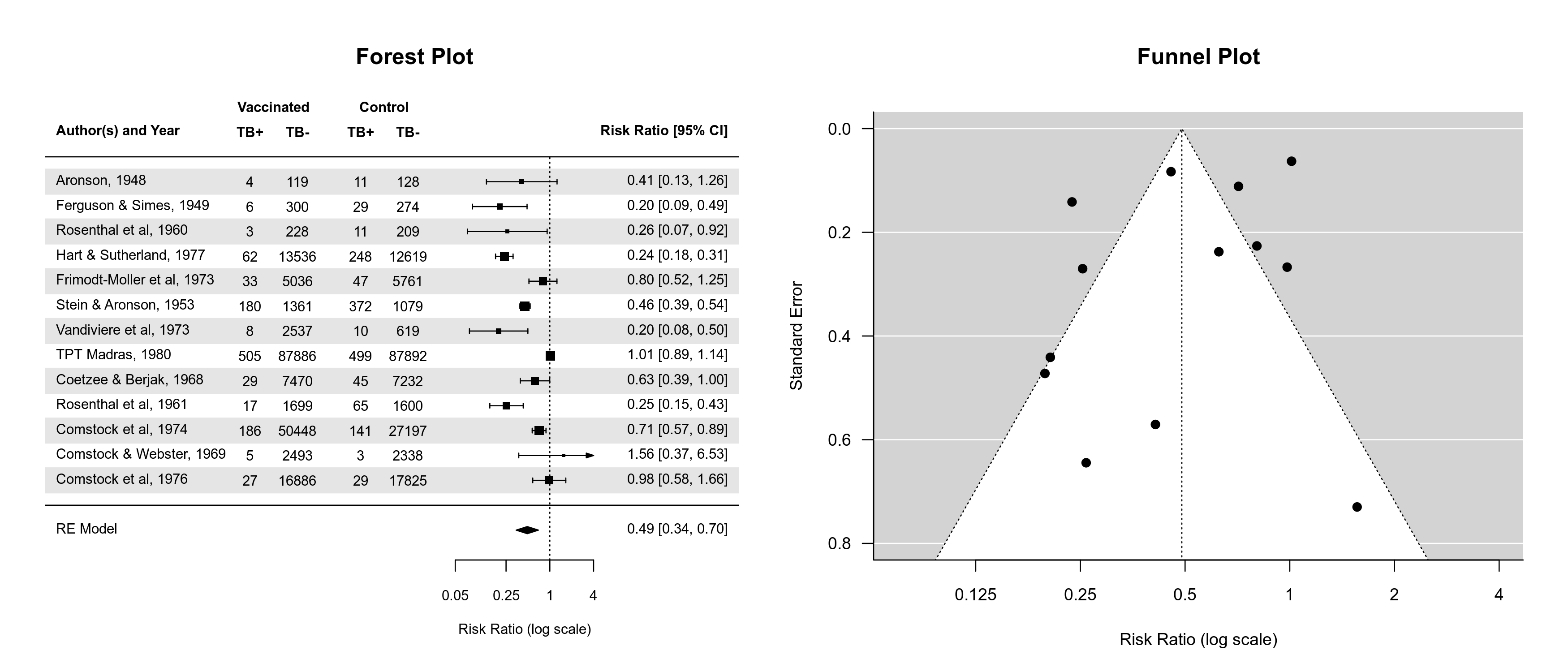#The metafor Package

A Meta-Analysis Package for R

news:news

## Package News

### 2023-05-08: Version 4.2-0 Released on CRAN

I made a relatively quick release of another version of the package. There were a few minor little buglets that annoyed me that I wanted to get rid of right away. Along the way, I made some improvements to various plotting functions. In particular, the various forest() functions now do a better job of choosing default values for the xlim argument and the ilab.xpos argument values are now also automatically chosen when not specified (although it is still recommended to adjust the default values to tweak the look of each forest plot into perfection). There is now also a shade argument for shading particular rows of the plot (e.g., for a 'zebra-like' look).

Also, the various plotting functions now respect par("fg"). This makes it possible to easily create plots with a dark background and light plotting colors. By default, plots created in R have a light (white) background and use dark colors, like this:But if we set up the plotting device like this:

bg <- "gray10"
fg <- "gray95"
dev.new(canvas=bg)
par(fg=fg, bg=bg, col=fg, col.axis=fg, col.lab=fg, col.main=fg, col.sub=fg)

then the resulting plots look like this:So in case you prefer a dark mode for your IDE/editor, opening a plot in this manner no longer feels like starring directly into the sun.Such an awesome movie, by the way.

Finally, aside from a few other improvements (e.g., functions that issue a warning when omitting studies due to NAs now indicate how many were omitted), the rma.glmm() function (when measure="OR", model="CM.EL", method="ML") now treats $\tau^2$ values below 1e-04 effectively as zero before computing the standard errors of the fixed effects. This helps to avoid numerical problems in approximating the Hessian. Similarly, selmodel() now treats $\tau^2$ values below 1e-04 or min(vi/10) effectively as zero before computing the standard errors.

The full changelog can be found here.

### 2023-03-19: Version 4.0-0 Released on CRAN

I am excited to announce the official (i.e., CRAN) release of version 4.0-0 of the metafor package. This will be the 30th update to the package after its initial release in 2009. Since then, the package has grown from a measly 4460 lines of code / 60 functions / 76 pages of documentation to a respectable 36879 lines of code / 330 functions / 347 pages of documentation. Aside from a few improvements related to modeling (e.g., the emmprep() function provides easier interoperability with the emmeans package and the selmodel() function gains a few additional selection models), I would say the focus of this update was on steps that occur prior to modeling, namely the calculation of the chosen effect size measure (or outcome measure as I prefer to call it) and the construction of the dataset in general.

In particular, the escalc() function now allows the user to also input appropriate test statistics and/or p-values for a number of measures where these can be directly transformed into the corresponding values of the measure. For example, the t-statistic from an independent samples t-test can be easily transformed into a standardized mean difference or the t-statistic from a standard test of a correlation coefficient can be easily transformed into the correlation coefficient or its r-to-z transformed version. Speaking of the latter, essentially all correlation-type measures can now be transformed using the r-to-z transformation, although it should be noted that this is not a proper variance-stabilizing transformation for all measures. This can still be useful though since the r-to-z transformation also has normalizing properties and when combining different types of correlation coefficients in the same analysis (e.g., Pearson product-moment correlations and tetrachoric/biserial correlations).

Finally, there are now several functions in the package that facilitate the construction of the dataset for a meta-analysis more generally. The conv.2x2() function helps to reconstruct 2x2 tables based on various pieces of information (e.g., odds ratios, chi-square statistics), while the conv.fivenum() function provides various methods for computing (or more precisely, estimating) means and standard deviations based on five-number summary values (i.e., the minimum, first quartile, median, third quartile, and maximum) and subsets thereof. The conv.wald() function converts Wald-type tests and/or confidence intervals to effect sizes and corresponding sampling variances (e.g., to transform a reported odds ratio and its confidence interval to the corresponding log odds ratio and sampling variance). And the conv.delta() function transforms effect sizes or outcomes and their sampling variances using the delta method, which can be useful in several data preparations steps. See the documentation of these functions for further details and examples.

If you come across any issues/bugs, please report them here. However, for questions or discussions about these functions (or really anything related to the metafor package or meta-analysis with R in general), please use the R-sig-meta-analysis mailing list.